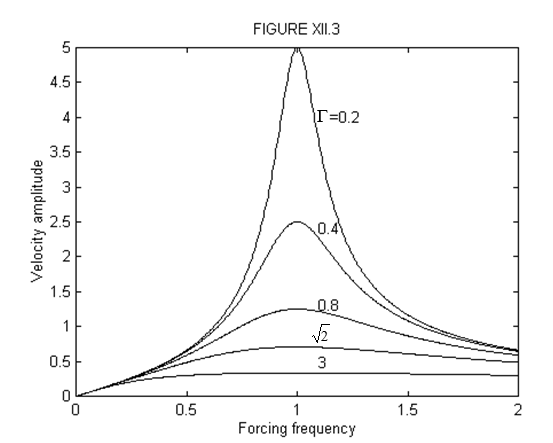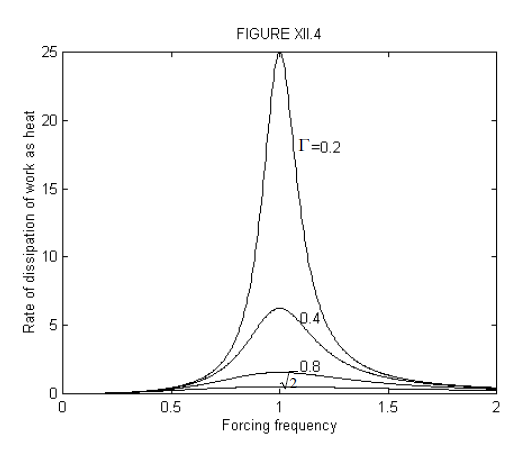$$\require{cancel}$$

12.2: Forced Oscillatory Motion

•• Contributed by Jeremy Tatum
• Emeritus Professor (Physics & Astronomy) at University of Victoria

We are thinking of a mass $$m$$ attached to a spring of force constant $$k$$ and subject to a damping force $$b\dot{x}$$, but also subject to a periodic sinusoidal force $$\hat{F} \cos \omega t$$. The equation of motion is

$m\ddot{x}+b\dot{x}+kx=\hat{F} \cos \omega t, \label{12.2.1}$

or, if we divide by $$m$$:

$\ddot{x}+\gamma \dot{x}+\omega_{0}^{2}=\hat{f}\cos\omega t. \label{12.2.2}$

Here $$\gamma = \frac{b}{m},\quad \omega_{0}^{2}=\frac{k}{m},\quad \hat{f}=\frac{\hat{F}}{m}$$. $$\omega$$ is the forcing angular frequency and $$\omega_{0}$$ is the natural frequency of mass and spring in the absence of damping. One part of the general solution of Equation $$\ref{12.2.2}$$ is the complementary function, which we have dealt with at length in Chapter 11. In this section I shall be interested in the particular integral. I shall not derive it here (those who are familiar with differential equations will be able to do so), but you should at least verify by differentiation and substitution that the following is a solution, and it is indeed the particular integral:

$x=\frac{\hat{f}}{(\omega_{0}^{2}-\omega^{2})^{2}+\gamma^{2}\omega^{2}} \left[(\omega_{0}^{2}-\omega^{2})\cos\omega t+\gamma\omega\sin\omega t\right]. \label{12.2.3}$

This can also be written

$x=\frac{\hat{f}}{[(\omega_{0}^{2}-\omega^{2})^{2}+\gamma^{2}\omega^{2}]^{\frac{1}{2}}}\cos(\omega t-\alpha), \label{12.2.4}$

where

$\cos\alpha=\frac{\omega_{0}^{2}-\omega^{2}}{[(\omega_{0}^{2}-\omega^{2})^{2}+\gamma^{2}\omega^{2}]^{\frac{1}{2}}},\quad \cos\alpha=\frac{\gamma\omega}{[(\omega_{0}^{2}-\omega^{2})^{2}+\gamma^{2}\omega^{2}]^{\frac{1}{2}}},\quad \tan\alpha=\frac{\gamma \omega}{\omega_{0}^{2}-\omega^{2}}. \label{12.2.5}$

The response frequency is the same as the forcing frequency, but there is a phase lag between $$x$$ and $$F$$. Figure XII.1 shows $$\alpha$$ as a function of $$\Omega=\frac{\omega}{\omega_{0}}$$ for several different values of $$\Gamma=\frac{\gamma}{\omega_{0}}$$.

The particular integral can also be written

$x=\hat{x}\cos(\omega t-\alpha), \label{12.2.6}$

where the displacement amplitude $$\hat{x}$$ varies with forcing frequency $$\omega$$ as

$\hat{x}=\frac{\hat{f}}{[(\omega_{0}^{2}-\omega^{2})^{2}+\gamma^{2}\omega^{2}]^{\frac{1}{2}}}. \label{12.2.7}$

If we now introduce dimensionless quantities

$\Omega=\frac{\omega}{\omega_{0}},\quad \Gamma=\frac{\gamma}{\omega_{0}},\quad \hat{X}=\frac{\hat{x}}{\frac{\hat{f}}{\omega_{0}^{2}}}, \label{12.2.8}$

Equations $$\ref{12.2.5}$$ and $$\ref{12.2.7}$$ become

$\tan\alpha=\frac{\Gamma\Omega}{1-\Omega^{2}} \label{12.2.9}$

and

$\hat{X}=\frac{1}{[(1-\Omega^{2})^{2}+\Gamma^{2}\Omega^{2}]^{\frac{1}{2}}}. \label{12.2.10}$

The phase lag $$\alpha$$ and the displacement amplitude $$\hat{X}(=\frac{\hat{x}}{(\frac{\hat{f}}{\omega_{0}^{2}})})$$ are shown as a function of forcing frequency for various values of the damping constant in figures XII.1 and 2.A common misunderstanding is that the displacement amplitude is greatest when the forcing frequency is equal to the undamped frequency $$\omega_{0}$$. That this is far from the case is immediately obvious from a glance at figure XII.2. We can find the frequency that results in the greatest displacement amplitude by maximizing Equation $$\ref{12.2.7}$$ or $$\ref{12.2.10}$$. This is most easily achieved by minimizing the square of the denominator. Let $$D$$ be the square of the denominator of equation $$\ref{12.2.10}$$, and let $$W=\Omega^{2}$$ and $$G=\Gamma^{2}$$. Then $$D=(1-W)^{2}+GW$$, which is greatest for $$W=1-\frac{1}{2}G$$ or, provided $$\gamma < \sqrt{2}\omega_{0}$$

$\omega=\sqrt{\omega_{0}^{2}-\frac{1}{2}\gamma^{2}}. \label{12.2.11}$

This is less not only than $$\omega_{0}$$, but also less than $$\omega^{'}$$. For the frequency given by equation $$\ref{12.2.11}$$, the displacement amplitude will be

$\hat{x}_{max}=\frac{\hat{f}}{\gamma(\omega_{0}^{2}-\frac{1}{4}\gamma^{2})^{\frac{1}{2}}}. \label{12.2.12}$The locus of the maxima in figure XII.2 is found by eliminating $$\gamma$$ from equation 12.2.7 and $$\frac{d\hat{x}}{d\omega}=0$$ which gives

$\hat{x}_{max}=\frac{\hat{f}}{\sqrt{\omega_{0}^{4}-\omega^{4}}}. \label{12.2.13}$

The solution given by Equations $$\ref{12.2.7}$$ and $$\ref{12.2.8}$$ is the particular integral. As pointed out in Section 1 of this chapter, the complete solution is the sum of the particular integral and the complementary function, the latter being the unforced solutions of Chapter 11. The particular integral represents the steady state solution, whereas the complementary function, which dies out with time, is a transient solution. When a mechanical oscillation is started, or when an alternating current electric circuit is first switched on, the solution is the sum of transient and steady state parts, the former more or less rapidly dying away. Often when an electric fuse blows, the overload is caused by the large, but temporary, amplitude of the transient part of the solution.

Equations $$\ref{12.2.7}$$ and $$\ref{12.2.8}$$ give the displacement of the system as a function of time. Differentiation with respect to time gives the velocity as a function of time. Thus:

$\dot{x}=v=-\hat{v}\sin(\omega t-\alpha), \label{12.2.14}$

where

$\hat{v}=\frac{\hat{f}\omega}{[(\omega_{0}^{2}-\omega^{2})^{2}+\gamma^{2}\omega^{2}]^{\frac{1}{2}}} \label{12.2.15}$

is the velocity amplitude. In dimensionless units, this can be written

$\hat{V}=\frac{\Omega}{[(1-\Omega^{2})^{2}+\Gamma^{2}\Omega^{2}]^{\frac{1}{2}}}, \label{12.2.16}$

where

$\hat{V}=\frac{\hat{v}}{\frac{\hat{f}}{\omega_{0}}}. \label{12.2.17}$

This is illustrated in figure XII.3.It is left to the reader to show that the velocity amplitude is greatest and equal to $$\frac{\hat{f}}{\gamma}$$ when $$\omega=\omega_{0}$$.

We have now found the phase lag and the displacement and velocity amplitudes as a function of forcing frequency, but I must now try the reader's patience one step further for the most important part of the analysis, which really must not be skipped. Damping of oscillatory motion implies that some of the mechanical energy (which, in an undamped system, alternates between kinetic and potential energy) is lost - or, rather, that it is dissipated as heat. This happens if the damping is caused by the oscillator being immersed in a viscous fluid, or if it is caused by the repeated expansion and compression of a spring, or, in an electric circuit, by the dissipation of heat in the resistive part of the circuit. We aim now to find the rate at which the mechanical energy is dissipated as heat.

We return to the equation of motion, Equation $$\ref{12.2.1}$$:

$$m\ddot{x}+b\dot{x}+kx=\hat{F}\cos\omega t$$

and multiply each side by $$\dot{x}$$:

$m\dot{x}\ddot{x}+b\dot{x}^{2}+kx\dot{x}=\dot{x}\hat{F}\cos\omega t. \label{12.2.18}$

Introduce the total mechanical energy:

$E=\frac{1}{2}m\dot{x}^{2}+\frac{1}{2}kx^{2}. \label{12.2.19}$

The instantaneous rate of change of $$E$$ is $$m\dot{x}\ddot{x}+kx\dot{x}$$ while the instantaneous rate at which $$F$$ does work is $$\dot{x}F\cos\omega t$$. The difference (see Equation $$\ref{12.2.18}$$), $$b\dot{x}^{2}$$, is therefore the rate at which work is being dissipated as heat, which, of course, is zero if $$b=0$$.

The average of $$b\dot{x}^{2}$$ over a complete period is

$\overline{b\dot{x}^{2}}=\frac{b\hat{f}^{2}\omega^{2}}{(\omega_{0}^{2}-\omega^{2})+\gamma^{2}\omega^{2}}\overline{\sin^{2}(\omega t-\alpha)} \label{12.2.20}$

where the bars denote the average value over a period. But $$\overline{\sin^{2}(\omega t-\alpha)}=\frac{1}{2}$$, so the average rate at which work is being dissipated as heat, for which I shall use the symbol $$\dot{Q}$$ is

$\dot{Q}=\frac{b\hat{f}^{2}\omega^{2}}{2[(\omega_{0}^{2}-\omega^{2})^{2}+\gamma^{2}\omega^{2}]} \label{12.2.21}$

The reader should check that the right hand side has the dimensions of rate of dissipation of energy and hence the SI unit of watts.

In dimensionless units, in which $$\dot{Q}^{*}=\frac{\dot{Q}}{(\frac{b\hat{f}^{2}}{2\omega_{0}})}$$ this can be written

$\dot{Q}^{*}=\frac{\Omega^{2}}{(1-\Omega^{2})^{2}+\Gamma^{2}\Omega^{2}}. \label{12.2.22}$

This is illustrated in figure XII.4. The reader can easily prove that the rate at which work is dissipated as heat is greatest when the forcing frequency is equal to $$\omega_{0}$$.Summary

Phase lag: Equation $$\ref{12.2.5}$$

$$\cos\alpha=\frac{\omega_{0}^{2}-\omega^{2}}{[(\omega_{0}^{2}-\omega^{2})^{2}+\gamma^{2}\omega^{2}]^{\frac{1}{2}}},\quad \cos\alpha=\frac{\gamma\omega}{[(\omega_{0}^{2}-\omega^{2})^{2}+\gamma^{2}\omega^{2}]^{\frac{1}{2}}},\quad \tan\alpha=\frac{\gamma \omega}{\omega_{0}^{2}-\omega^{2}}$$.

Displacement amplitude: Equation $$\ref{12.2.7}$$

$$\hat{x}=\frac{\hat{f}}{[(\omega_{0}^{2}-\omega^{2})^{2}+\gamma^{2}\omega^{2}]^{\frac{1}{2}}}$$

Velocity amplitude: Equation $$\ref{12.2.15}$$

$$\hat{v}=\frac{\hat{f}\omega}{[(\omega_{0}^{2}-\omega^{2})^{2}+\gamma^{2}\omega^{2}]^{\frac{1}{2}}}$$

Rate of dissipation of work as heat: Equation $$\ref{12.2.21}$$

$$\dot{Q}=\frac{m\gamma\hat{f}^{2}\omega^{2}}{2[(\omega_{0}^{2}-\omega^{2})^{2}+\gamma^{2}\omega^{2}]}$$.

In terms of dimensionless variables,

Phase lag: Equation $$\ref{12.2.9}$$

$$\tan\alpha=\frac{\Gamma\Omega}{1-\Omega^{2}}$$

Displacement amplitude: Equation $$\ref{12.2.10}$$

$$\hat{X}=\frac{1}{[(1-\Omega^{2})^{2}+\Gamma^{2}\Omega^{2}]^{\frac{1}{2}}}$$

Velocity amplitude: $$\ref{12.2.16}$$

$$\hat{V}=\frac{\Omega}{[(1-\Omega^{2})^{2}+\Gamma^{2}\Omega^{2}]^{\frac{1}{2}}}$$

Rate of dissipation of work as heat: $$\ref{12.2.22}$$

$$\dot{Q}^{*}=\frac{\Omega^{2}}{(1-\Omega^{2})^{2}+\Gamma^{2}\Omega^{2}}$$